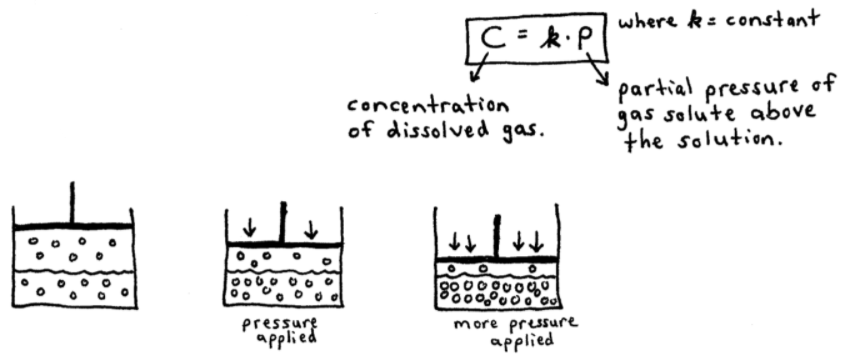# S11E2 - Three Factors Affecting the Solubility of a Solution

There are 3 factors that must be considered when determining the solubility of a solution. They are:

1. Structure Effects
2. Pressure Effects
3. Temperature

Let's take a look at each of these factors in detail.

## 1. Structure Effects

➞ Structure Effects  =  "like dissolves like"

Solubility is favored when the solute and the solvent have similar properties. In other words:

- polar solutes dissolve in polar solvents.
- nonpolar solutes dissolve in nonpolar solvents.

ex:  vitamins A, D, E, and K are fat-soluble, while vitamins B and C are water-soluble.

-----

## 2. Pressure Effects

This only affects gas solubility in a solvent!

ex:  carbonation, CO2(g), in a bottle of soda...

➞ To ensure a large amount of CO2 is dissolved in water, soda is bottled at high pressures. "Fizzing" occurs as CO2(g) molecules escape from the liquid solvent because they're no longer soluble at atmospheric pressure (1 atm).

### Henry's Law

Henry's Law = the amount of gas dissolved in a solution is proportional to the pressure of the gas above the solution:

C = kP  ,  where k = constant

C = concentration of the dissolved gas
P = partial pressure of the gas solute above the solution-----

## 3. Temperature Effects

We'll assume our solvent is H2O and thus all solutions are "aqueous solutions."

Let's talk about the solubilities of solid, liquid, and gas solutes separately...

a. solid solute  (ex: sugar)

➞ most solids become more soluble as temperature increases.

b. liquid solute  (ex: ethanol)

➞ most liquids become more soluble as temperature increases.

c. gas solute  (ex: CO2)

➞ most gas solutes become less soluble as temperature increases.
Why?...

Because as the temperature of the solution increases, dissolved gas particles have a greater tendency to escape the liquid, thus decreasing their solubility in the solvent.

K.E. ∝ T

If the temperature increases, the kinetic energy of the moving gas particles also increases. More energy means greater tendency to escape the H2O solvent.

=====

Next up in SECTION 11 - Solutions and Their Properties,

We'll talk about the Vapor Pressure of Solutions, Nonvolatile Solutes, and Raoult's Law.